### 2 精确率与召回率

#### 2.1 准确率弊端

$\text{Accuracy}=\frac{99900+5}{100000+200}\approx 0.997\phantom{\rule{0.278em}{0ex}}\phantom{\rule{0.278em}{0ex}}\phantom{\rule{0.278em}{0ex}}\phantom{\rule{0.278em}{0ex}}\phantom{\rule{0.278em}{0ex}}\phantom{\rule{0.278em}{0ex}}\phantom{\rule{0.278em}{0ex}}\phantom{\rule{0.278em}{0ex}}\phantom{\rule{0.278em}{0ex}}\left(1\right)$

#### 2.2 精确率与召回率原理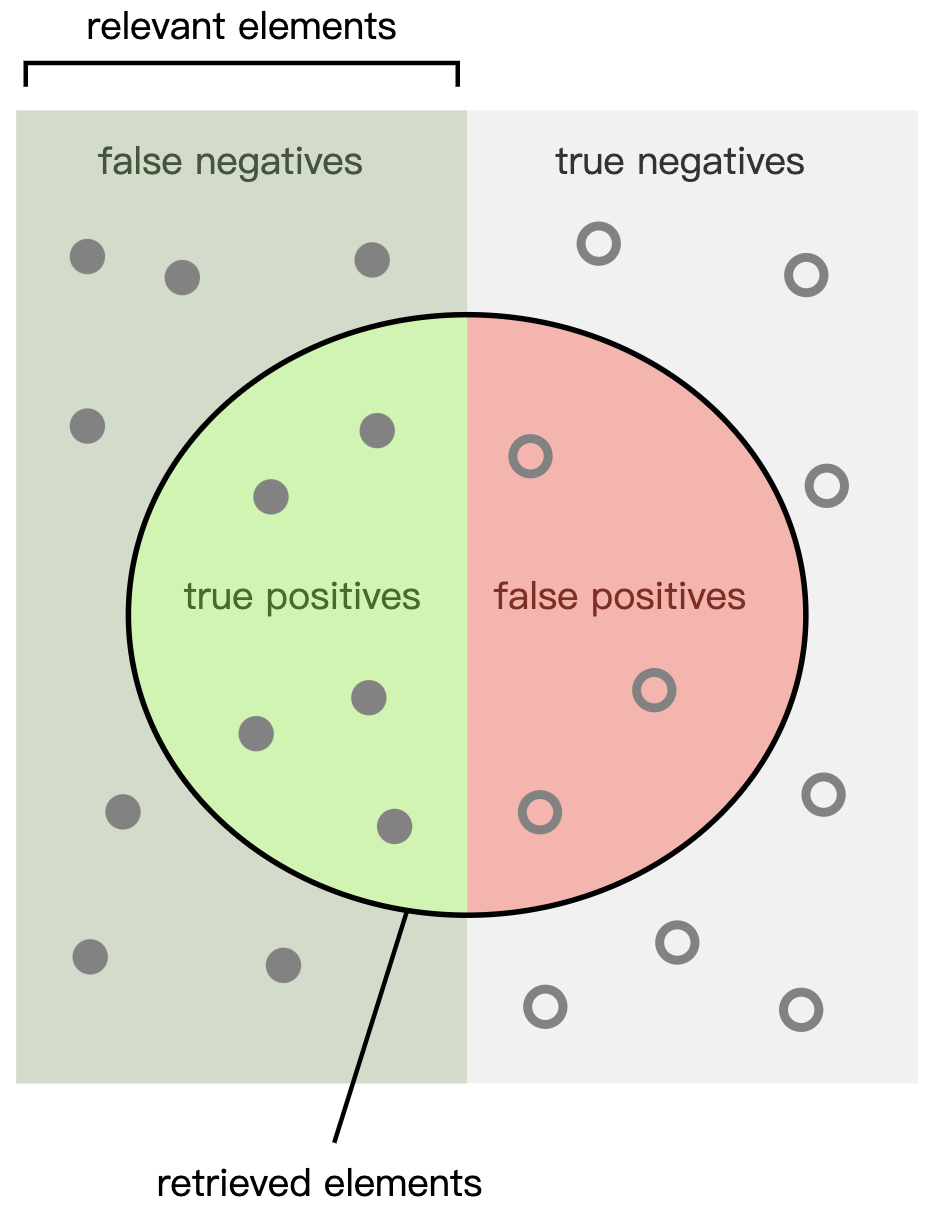$\text{Precision}=\frac{\text{TP}}{\text{TP}+\text{FP}}=\frac{5}{5+3}=\frac{5}{8}\phantom{\rule{0.278em}{0ex}}\phantom{\rule{0.278em}{0ex}}\phantom{\rule{0.278em}{0ex}}\phantom{\rule{0.278em}{0ex}}\phantom{\rule{0.278em}{0ex}}\phantom{\rule{0.278em}{0ex}}\phantom{\rule{0.278em}{0ex}}\left(2\right)$

$\text{Recall}=\frac{\text{TP}}{\text{TP}+\text{FN}}=\frac{5}{5+7}=\frac{5}{12}\phantom{\rule{0.278em}{0ex}}\phantom{\rule{0.278em}{0ex}}\phantom{\rule{0.278em}{0ex}}\phantom{\rule{0.278em}{0ex}}\phantom{\rule{0.278em}{0ex}}\phantom{\rule{0.278em}{0ex}}\phantom{\rule{0.278em}{0ex}}\left(3\right)$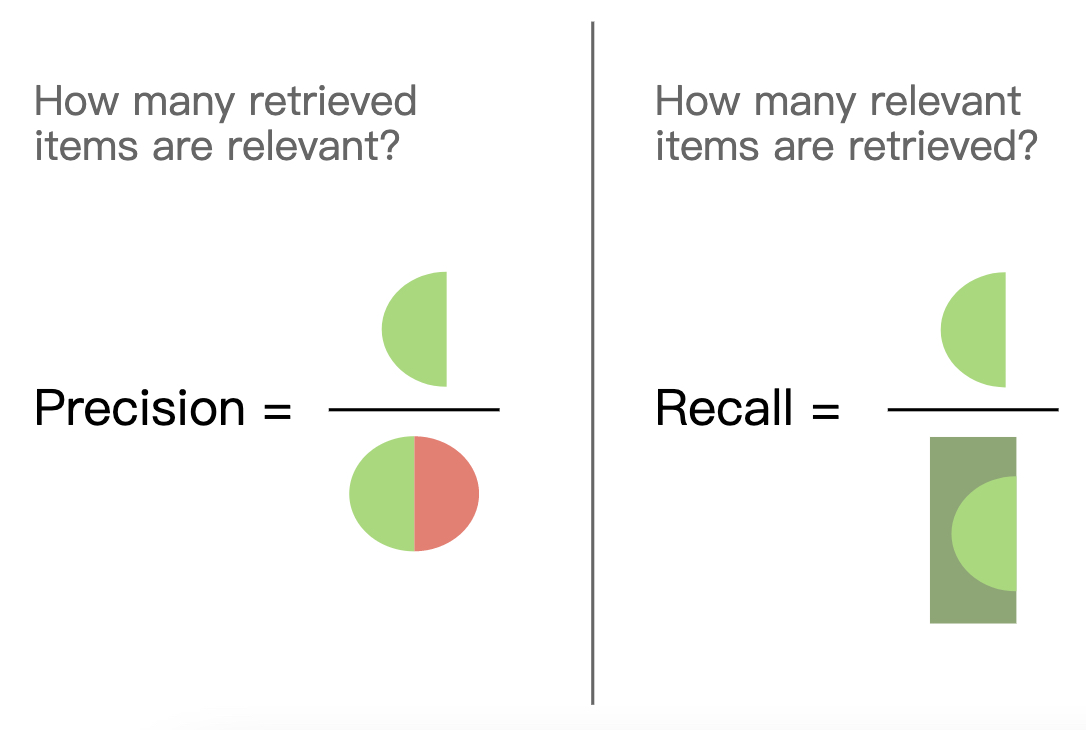• TP（表示将正样本预测为正样本，即预测正确）为：$5$$5$
• FN（表示将正样本预测为负样本，即预测错误）为：$195$$195$
• FP（表示将负样本预测为正样本，即预测错误）为：$100$$100$
• TN（表示将负样本预测为负样本，即预测正确）为：$99900$$99900$

$\begin{array}{rl}\text{Accuracy}& =\frac{\text{TP}+\text{TN}}{\text{TP}+\text{TN}+\text{FP}+\text{FN}}=\frac{99905}{100200}\approx 0.997\\ \text{Precision}& =\frac{\text{TP}}{\text{TP}+\text{FP}}=\frac{5}{5+100}\approx 0.05\phantom{\rule{0.278em}{0ex}}\phantom{\rule{0.278em}{0ex}}\phantom{\rule{0.278em}{0ex}}\phantom{\rule{0.278em}{0ex}}\phantom{\rule{0.278em}{0ex}}\phantom{\rule{0.278em}{0ex}}\phantom{\rule{0.278em}{0ex}}\phantom{\rule{0.278em}{0ex}}\phantom{\rule{0.278em}{0ex}}\phantom{\rule{0.278em}{0ex}}\phantom{\rule{0.278em}{0ex}}\phantom{\rule{0.278em}{0ex}}\phantom{\rule{0.278em}{0ex}}\phantom{\rule{0.278em}{0ex}}\phantom{\rule{0.278em}{0ex}}\phantom{\rule{0.278em}{0ex}}\phantom{\rule{0.278em}{0ex}}\phantom{\rule{0.278em}{0ex}}\phantom{\rule{0.278em}{0ex}}\phantom{\rule{0.278em}{0ex}}\phantom{\rule{0.278em}{0ex}}\phantom{\rule{0.278em}{0ex}}\phantom{\rule{0.278em}{0ex}}\left(4\right)\\ \text{Recall}& =\frac{\text{TP}}{\text{TP}+\text{FN}}=\frac{5}{5+195}=0.025\end{array}$

#### 2.3 准确率与召回率区别

$\begin{array}{rl}\text{Accuracy}& =\frac{200}{100200}\approx 0.002\\ \text{Precision}& =\frac{200}{100000+200}\approx 0.002\phantom{\rule{0.278em}{0ex}}\phantom{\rule{0.278em}{0ex}}\phantom{\rule{0.278em}{0ex}}\phantom{\rule{0.278em}{0ex}}\phantom{\rule{0.278em}{0ex}}\phantom{\rule{0.278em}{0ex}}\phantom{\rule{0.278em}{0ex}}\phantom{\rule{0.278em}{0ex}}\phantom{\rule{0.278em}{0ex}}\phantom{\rule{0.278em}{0ex}}\phantom{\rule{0.278em}{0ex}}\phantom{\rule{0.278em}{0ex}}\phantom{\rule{0.278em}{0ex}}\phantom{\rule{0.278em}{0ex}}\phantom{\rule{0.278em}{0ex}}\phantom{\rule{0.278em}{0ex}}\phantom{\rule{0.278em}{0ex}}\left(5\right)\\ \text{Recall}& =\frac{200}{200+0}=1.\end{array}$

$\begin{array}{rl}\text{Accuracy}& =\frac{50+100000}{100200}\approx 0.999\\ \text{Precision}& =\frac{50}{50+0}=1.0\phantom{\rule{0.278em}{0ex}}\phantom{\rule{0.278em}{0ex}}\phantom{\rule{0.278em}{0ex}}\phantom{\rule{0.278em}{0ex}}\phantom{\rule{0.278em}{0ex}}\phantom{\rule{0.278em}{0ex}}\phantom{\rule{0.278em}{0ex}}\phantom{\rule{0.278em}{0ex}}\phantom{\rule{0.278em}{0ex}}\phantom{\rule{0.278em}{0ex}}\phantom{\rule{0.278em}{0ex}}\phantom{\rule{0.278em}{0ex}}\phantom{\rule{0.278em}{0ex}}\phantom{\rule{0.278em}{0ex}}\phantom{\rule{0.278em}{0ex}}\phantom{\rule{0.278em}{0ex}}\phantom{\rule{0.278em}{0ex}}\phantom{\rule{0.278em}{0ex}}\phantom{\rule{0.278em}{0ex}}\phantom{\rule{0.278em}{0ex}}\phantom{\rule{0.278em}{0ex}}\phantom{\rule{0.278em}{0ex}}\left(6\right)\\ \text{Recall}& =\frac{50}{50+150}=0.25\end{array}$

$\begin{array}{rl}\text{Accuracy}& =\frac{190+99980}{100200}\approx 0.999\\ \text{Precision}& =\frac{190}{190+20}\approx 0.905\phantom{\rule{0.278em}{0ex}}\phantom{\rule{0.278em}{0ex}}\phantom{\rule{0.278em}{0ex}}\phantom{\rule{0.278em}{0ex}}\phantom{\rule{0.278em}{0ex}}\phantom{\rule{0.278em}{0ex}}\phantom{\rule{0.278em}{0ex}}\phantom{\rule{0.278em}{0ex}}\phantom{\rule{0.278em}{0ex}}\phantom{\rule{0.278em}{0ex}}\phantom{\rule{0.278em}{0ex}}\phantom{\rule{0.278em}{0ex}}\phantom{\rule{0.278em}{0ex}}\phantom{\rule{0.278em}{0ex}}\phantom{\rule{0.278em}{0ex}}\phantom{\rule{0.278em}{0ex}}\phantom{\rule{0.278em}{0ex}}\phantom{\rule{0.278em}{0ex}}\left(7\right)\\ \text{Recall}& =\frac{190}{190+10}=0.95\end{array}$

$\text{F-score}=\left(1+{\beta }^{2}\right)\frac{\text{Precision}\cdot \text{Recall}}{{\beta }^{2}\cdot \left(\text{Precision}+\text{Recall}\right)}\phantom{\rule{0.278em}{0ex}}\phantom{\rule{0.278em}{0ex}}\phantom{\rule{0.278em}{0ex}}\phantom{\rule{0.278em}{0ex}}\phantom{\rule{0.278em}{0ex}}\phantom{\rule{0.278em}{0ex}}\phantom{\rule{0.278em}{0ex}}\phantom{\rule{0.278em}{0ex}}\phantom{\rule{0.278em}{0ex}}\left(8\right)$

$\text{F-score}=2\cdot \frac{\text{Precision}\cdot \text{Recall}}{\text{Precision}+\text{Recall}}\phantom{\rule{0.278em}{0ex}}\phantom{\rule{0.278em}{0ex}}\phantom{\rule{0.278em}{0ex}}\phantom{\rule{0.278em}{0ex}}\phantom{\rule{0.278em}{0ex}}\phantom{\rule{0.278em}{0ex}}\phantom{\rule{0.278em}{0ex}}\phantom{\rule{0.278em}{0ex}}\phantom{\rule{0.278em}{0ex}}\left(9\right)$

### 3 Precision-Recall 曲线

#### 3.1 Precision-Recall 曲线原理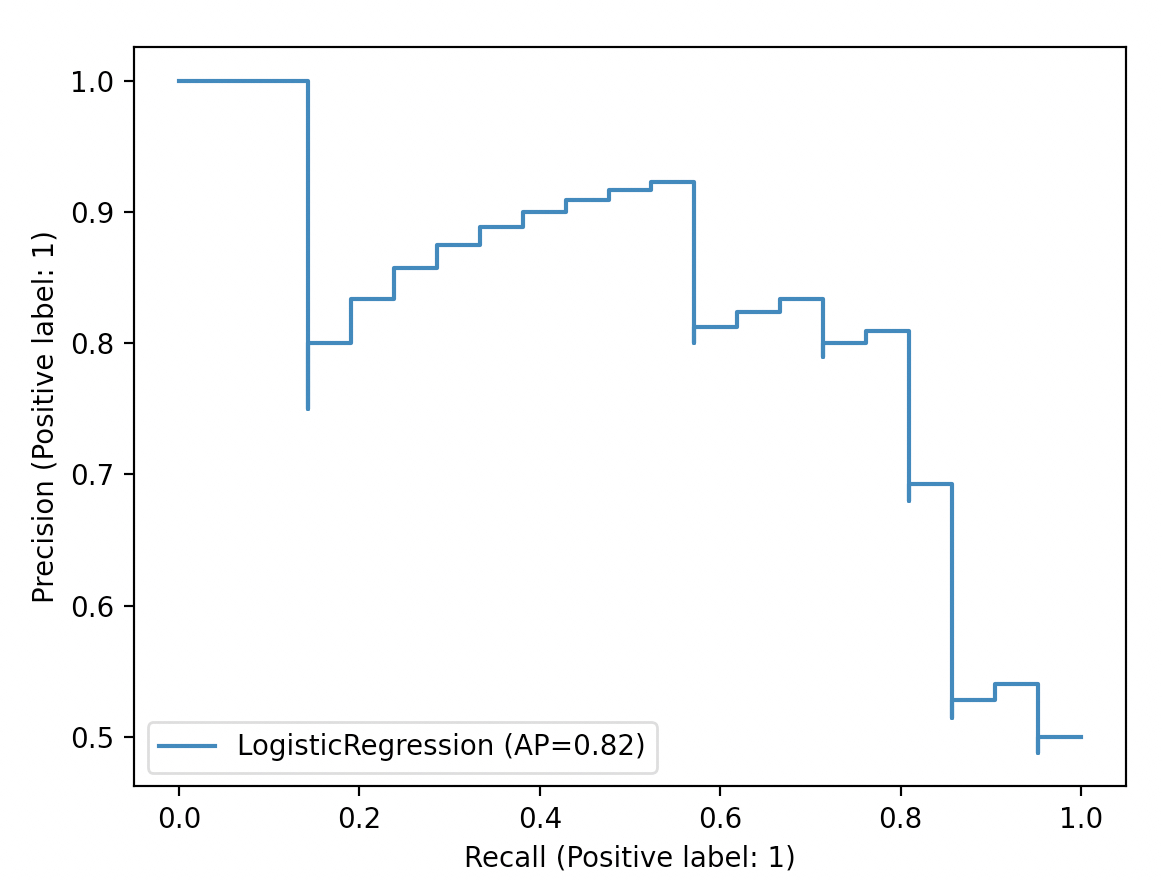#### 3.2 AUC计算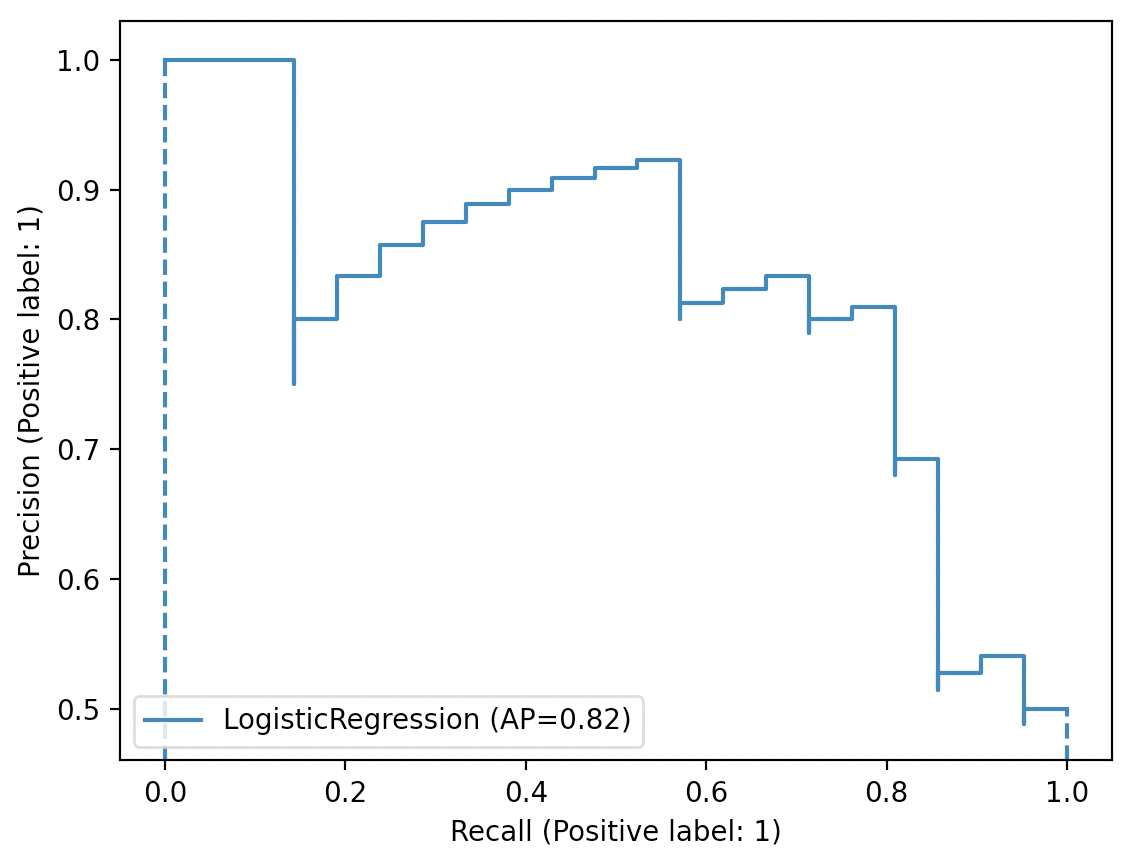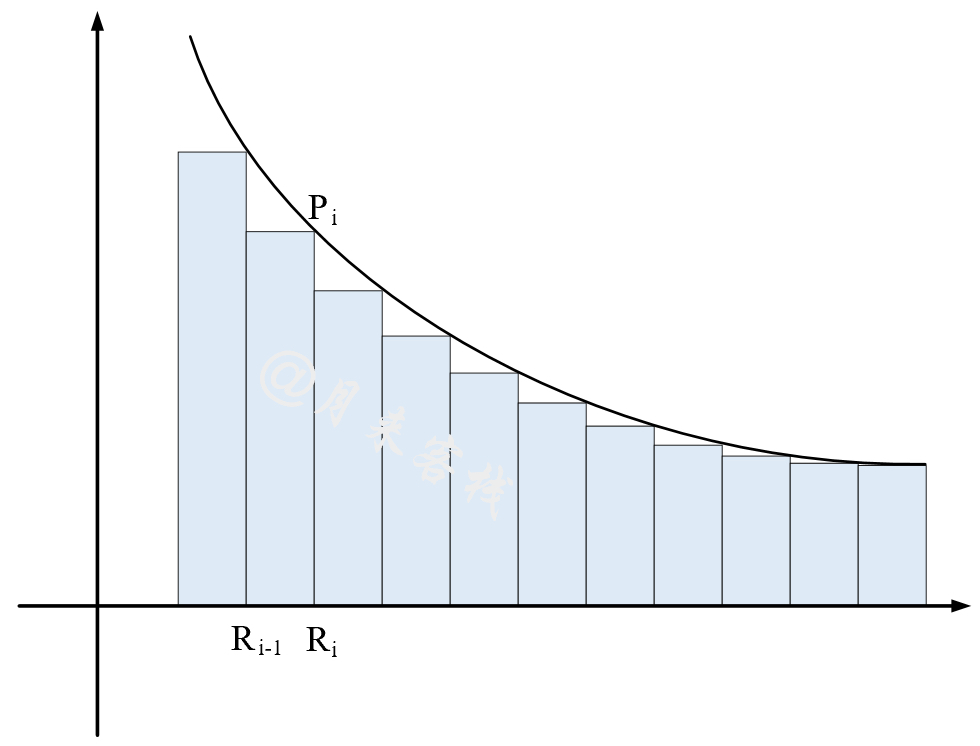$\text{AP}=\sum _{i}^{n}\left({R}_{i}-{R}_{i-1}\right){P}_{i}\phantom{\rule{0.278em}{0ex}}\phantom{\rule{0.278em}{0ex}}\phantom{\rule{0.278em}{0ex}}\phantom{\rule{0.278em}{0ex}}\phantom{\rule{0.278em}{0ex}}\phantom{\rule{0.278em}{0ex}}\phantom{\rule{0.278em}{0ex}}\phantom{\rule{0.278em}{0ex}}\phantom{\rule{0.278em}{0ex}}\left(10\right)$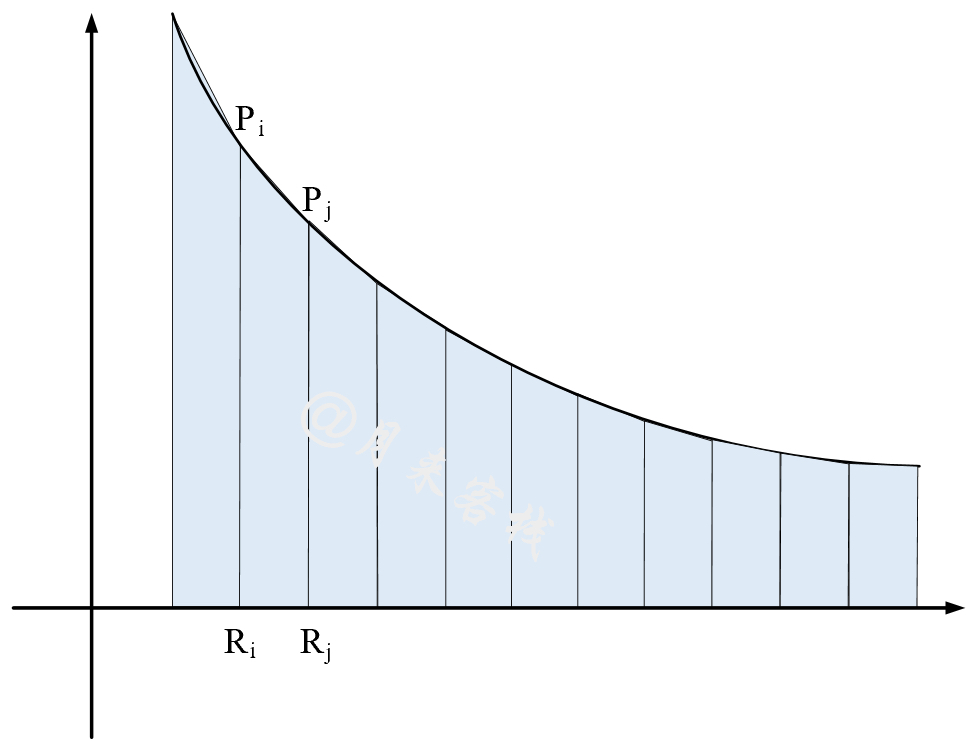$\text{AUC}=\sum _{i,j}^{n}\frac{1}{2}\left({P}_{i}+{P}_{j}\right)\left({R}_{j}-{R}_{i}\right),\phantom{\rule{0.278em}{0ex}}\phantom{\rule{0.278em}{0ex}}\left(i

### 4 代码实现

#### 4.1 指标计算

21def predict(y_scores, threholds):2    return (y_scores >= threholds) * 1

51from sklearn.metrics import precision_score, recall_score2def compute_scores(y_true, y_pred):3    p_score = precision_score(y_true, y_pred)4    r_score = recall_score(y_true, y_pred)5    return p_score, r_score

161def p_r_curve(y_true, y_scores):2    thresholds = sorted(np.unique(y_scores))3    precisions, recalls = [], []4    for thre in thresholds:5        y_pred = predict(y_scores, thre)6        r = compute_scores(y_true, y_pred)7        precisions.append(r)8        recalls.append(r)9    # 去掉召回率中末尾重复的情况10    last_ind = np.searchsorted(recalls[::-1], recalls) + 111    precisions = precisions[-last_ind:]12    recalls = recalls[-last_ind:]13    thresholds = thresholds[-last_ind:]14    precisions.append(1)15    recalls.append(0)16    return precisions, recalls, thresholds

161if __name__ == '__main__':2    y_true = np.array([0, 0, 0, 1, 1, 0, 1, 1])3    y_scores = np.array([0.5, 0.55, 0.74, 0.65, 0.28, 0.17, 0.3, 0.45])4    precision, recall, thresholds = precision_recall_curve(y_true, y_scores)5    ap = average_precision_score(y_true, y_scores)6    print("sklearn 计算结果：")7    print("precision", precision)8    print("recall", recall)9    print("thresholds", thresholds)10
11    print("\n编码实现 计算结果：")12    precision, recall, thresholds = p_r_curve(y_true, y_scores)13    ap = compute_ap(recall, precision)14    print("precision", precision)15    print("recall", recall)16    print("thresholds", thresholds)

91sklearn 计算结果：2precision [0.571428 0.5  0.4   0.25  0.333333  0.5  0. 1.]3recall [1.   0.75 0.5  0.25 0.25 0.25 0.   0.  ]4thresholds [0.28 0.3  0.45 0.5  0.55 0.65 0.74]5
6编码实现 计算结果：7precision [0.571428, 0.5, 0.4, 0.25, 0.333333, 0.5, 0.0, 1]8recall [1.0, 0.75, 0.5, 0.25, 0.25, 0.25, 0.0, 0]9thresholds [0.28, 0.3, 0.45, 0.5, 0.55, 0.65, 0.74]

81def compute_ap(recall, precision):2    # \\text{AP} = \\sum_n (R_n - R_{n-1}) P_n3    rp = [item for item in zip(recall, precision)][::-1]  # 按recall升序进行排序4    ap = 05    for i in range(1, len(rp)):6        ap += (rp[i] - rp[i - 1]) * rp[i]7        # print(f"({rp[i]} - {rp[i - 1]}) * {rp[i]}")8    return ap

111if __name__ == '__main__':2    y_true = np.array([0, 0, 0, 1, 1, 0, 1, 1])3    y_scores = np.array([0.5, 0.55, 0.74, 0.65, 0.28, 0.17, 0.3, 0.45])4    ap = average_precision_score(y_true, y_scores)5    print("sklearn 计算结果：")6    print("ap", ap) # ap 0.49285714285714297    print("auc", auc(recall, precision)) # auc 0.390178571428571438    9    print("\n编码实现 计算结果：")10    ap = compute_ap(recall, precision)11    print("ap", ap) # ap 0.4928571428571429

#### 4.2 结果可视化

111from sklearn.datasets import load_iris2from sklearn.model_selection import train_test_split3def get_dataset():4    x, y = load_iris(return_X_y=True)5    random_state = np.random.RandomState(2020)6    n_samples, n_features = x.shape7    x = np.concatenate([x, random_state.randn(n_samples, 200 * n_features)], axis=1)8    # 针对二分类下的pr曲线9    x_train, x_test, y_train, y_test = train_test_split(10                x[y < 2], y[y < 2], test_size=0.5, random_state=random_state)11    return x_train, x_test, y_train, y_test

xxxxxxxxxx161from sklearn.linear_model import LogisticRegression2if __name__ == '__main__':3    x_train, x_test, y_train, y_test = get_dataset()4    model = LogisticRegression()5    model.fit(x_train, y_train)6    y_scores = model.predict_proba(x_test)7    precision, recall, _ = p_r_curve(y_test, y_scores[:, 1])8    ap = compute_ap(recall, precision)9    plt.plot(recall, precision, drawstyle="steps-post", label=f'LogisticRegression (AP={ap})')10    plt.legend(loc="lower left")11    plt.xlabel("Recall (Positive label: 1)")12    plt.ylabel("Precision (Positive label: 1)")13    14    # 通过sklear方法进行绘制15    plot_precision_recall_curve(model, x_test, y_test)16    plt.show()

xxxxxxxxxx151if __name__ == '__main__':2    x_train, x_test, y_train, y_test = get_dataset()3    model = LogisticRegression(multi_class='ovr')4    model.fit(x_train, y_train)5    b_y = label_binarize(y_test, classes=[0, 1, 2])6    y_scores = model.predict_proba(x_test)7    for i in range(len(np.unique(y_test))):8        precision, recall, _ = p_r_curve(b_y[:, i], y_scores[:, i])9        ap = compute_ap(recall, precision)10        plt.plot(recall, precision, drawstyle="steps-post", 11                  label=f'Precision-recall for class {i} (AP = {ap})')12    plt.xlabel("Recall")13    plt.ylabel("Precision")14    plt.title("Precision-Recall curve by ours")15    plt.legend(loc="lower left")

xxxxxxxxxx121    # 通过sklear方法进行绘制2    _, ax = plt.subplots()3    for i in range(len(np.unique(y_test))):4        precision, recall, _ = p_r_curve(b_y[:, i], y_scores[:, i])5        ap = compute_ap(recall, precision)6        display = PrecisionRecallDisplay(7            recall=recall,8            precision=precision,9            average_precision=ap)10        display.plot(ax=ax, name=f"Precision-recall for class {i}")11    ax.set_title("Precision-Recall curve by sklearn")12    plt.show()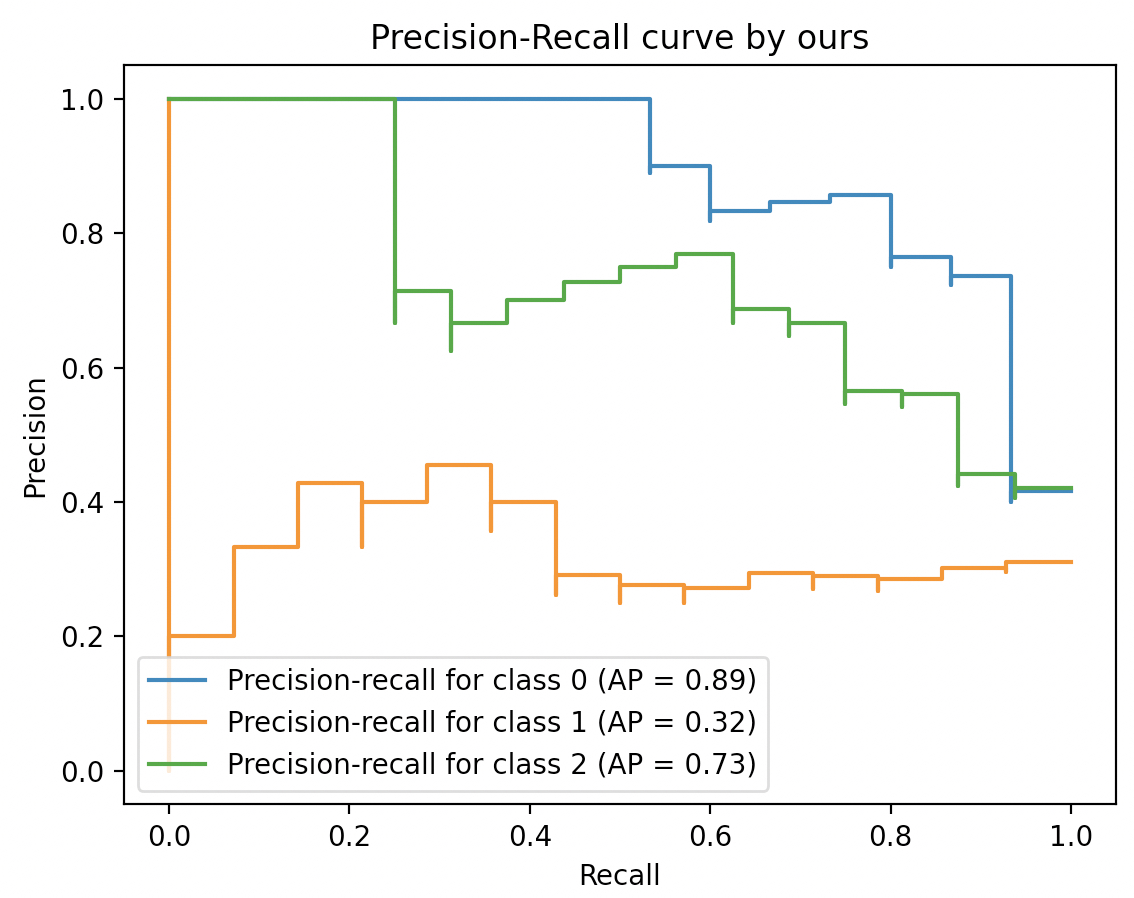### 引用

 多分类任务下的召回率与F值

 第3章从零认识逻辑回归（附高清PDF与教学PPT）